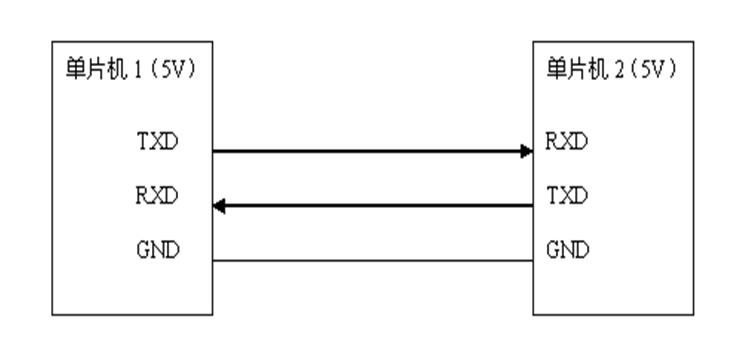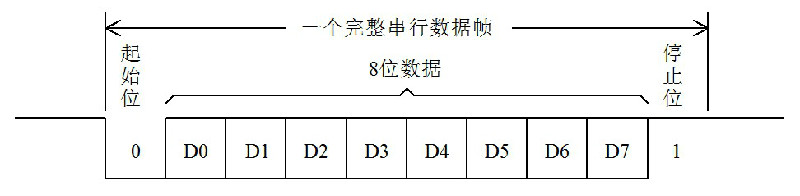# UART串口通信浅谈之(一)–基础概述

1.1 串行通信的初步认识如图1-2串口数据发送示意图，实际上是一个时域示意图，就是信号随着时间变化的对应关系。比如在单片机的发送引脚上，左边的是先发生的，右边的是后发生的，数据位的切换时间就是波特率分之一秒，如果能够理解时域的概念，后边很多通信的时序图就很容易理解了。

1.2 USB转串口通信CH340G这个电路很简单，把电源电路，晶振电路接好后，6脚和7脚的UD+和UD-分别接USB口的2个数据引脚上去，3脚和4脚接到了我们单片机的TXD和RXD上去。

CH340G的电路里2脚位置加了个4148的二极管，是一个小技巧。因为我们的STC89C52RC这个单片机下载程序需要冷启动，就是先点下载后上电，上电瞬间单片机会先检测需要不需要下载程序。虽然单片机的VCC是由开关来控制，但是由于CH340G的2脚是输出引脚，如果没有此二极管，开关后级单片机在断电的情况下，CH340G的2脚和单片机的P3.0（即RXD）引脚连在一起，有电流会通过这个引脚流入后级电路并且给后级的电容充电，造成后级有一定幅度的电压，这个电压值虽然只有两三伏左右，但是可能会影响到我们的冷启动。加了二极管后，一方面不影响通信，另外一个方面还可以消除这种问题。这个地方可以暂时作为了解，大家如果自己做这块电路，可以参考一下。

1.3 IO口模拟UART串口通信1. `#include <reg52.h>`

2. `sbit PIN_RXD = P3^0; //接收引脚定义`

3. `sbit PIN_TXD = P3^1; //发送引脚定义`

4. `bit RxdOrTxd = 0; //指示当前状态为接收还是发送`

5. `bit RxdEnd = 0; //接收结束标志`

6. `bit TxdEnd = 0; //发送结束标志`

7. `unsigned char RxdBuf = 0; //接收缓冲器`

8. `unsigned char TxdBuf = 0; //发送缓冲器`

9. `void ConfigUART(unsigned int baud);`

10. `void StartTXD(unsigned char dat);`

11. `void StartRXD();`

12. `void main ()`

13. `{`

14. `ConfigUART(9600); //配置波特率为9600`

15. `EA = 1; //开总中断`

16.
17. `while(1)`

18. `{`

19. `while (PIN_RXD); //等待接收引脚出现低电平，即起始位`

20. `StartRXD(); //启动接收`

21. `while (!RxdEnd); //等待接收完成`

22. `StartTXD(RxdBuf+1); //接收到的数据+1后，发送回去`

23. `while (!TxdEnd); //等待发送完成`

24. `}`

25. `}`

26. `void ConfigUART(unsigned int baud) //串口配置函数，baud为波特率`

27. `{`

28. `TMOD &= 0xF0; //清零T0的控制位`

29. `TMOD |= 0x02; //配置T0为模式2`

30. `TH0 = 256 - (11059200/12) / baud; //计算T0重载值`

31. `}`

32. `void StartRXD() //启动串行接收`

33. `{`

34. `TL0 = 256 - ((256-TH0) >> 1); //接收启动时的T0定时为半个波特率周期`

35. `ET0 = 1; //使能T0中断`

36. `TR0 = 1; //启动T0`

37. `RxdEnd = 0; //清零接收结束标志`

38. `RxdOrTxd = 0; //设置当前状态为接收`

39. `}`

40. `void StartTXD(unsigned char dat) //启动串行发送，dat为待发送字节数据`

41. `{`

42. `TxdBuf = dat; //待发送数据保存到发送缓冲器`

43. `TL0 = TH0; //T0计数初值为重载值`

44. `ET0 = 1; //使能T0中断`

45. `TR0 = 1; //启动T0`

46. `PIN_TXD = 0; //发送起始位`

47. `TxdEnd = 0; //清零发送结束标志`

48. `RxdOrTxd = 1; //设置当前状态为发送`

49. `}`

50. `void InterruptTimer0() interrupt 1 //T0中断服务函数，处理串行发送和接收`

51. `{`

52. `static unsigned char cnt = 0; //bit计数器，记录当前正在处理的位`

53. `if (RxdOrTxd) //串行发送处理`

54. `{`

55. `cnt++;`

56. `if (cnt <= 8) //低位在先依次发送8bit数据位`

57. `{`

58. `PIN_TXD = TxdBuf & 0x01;`

59. `TxdBuf >>= 1;`

60. `}`

61. `else if (cnt == 9) //发送停止位`

62. `{`

63. `PIN_TXD = 1;`

64. `}`

65. `else //发送结束`

66. `{`

67. `cnt = 0; //复位bit计数器`

68. `TR0 = 0; //关闭T0`

69. `TxdEnd = 1; //置发送结束标志`

70. `}`

71. `}`

72. `else //串行接收处理`

73. `{`

74. `if (cnt == 0) //处理起始位`

75. `{`

76. `if (!PIN_RXD) //起始位为0时，清零接收缓冲器，准备接收数据位`

77. `{`

78. `RxdBuf = 0;`

79. `cnt++;`

80. `}`

81. `else //起始位不为0时，中止接收`

82. `{`

83. `TR0 = 0; //关闭T0`

84. `}`

85. `}`

86. `else if (cnt <= 8) //处理8位数据位`

87. `{`

88. `RxdBuf >>= 1; //低位在先，所以将之前接收的位向右移`

89. `if (PIN_RXD) //接收脚为1时，缓冲器最高位置1；为0时不处理即仍保持移位后的0`

90. `{`

91. `RxdBuf |= 0x80;`

92. `}`

93. `cnt++;`

94. `}`

95. `else //停止位处理`

96. `{`

97. `cnt = 0; //复位bit计数器`

98. `TR0 = 0; //关闭T0`

99. `if (PIN_RXD) //停止位为1时，方能认为数据有效`

100. `{`

101. `RxdEnd = 1; //置接收结束标志`

102. `}`

103. `}`

104. `}`

105. `}`

THE END